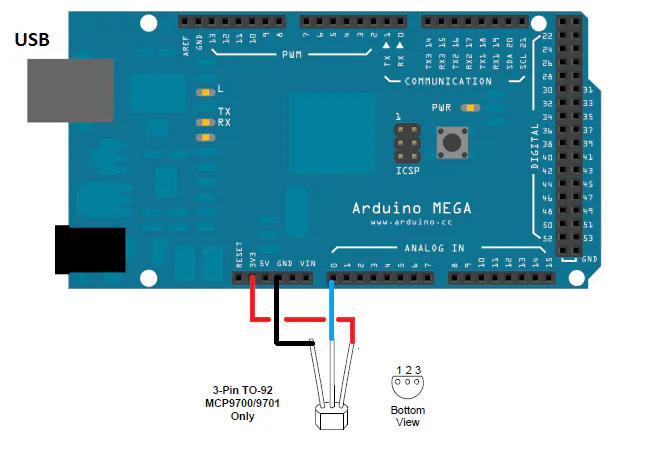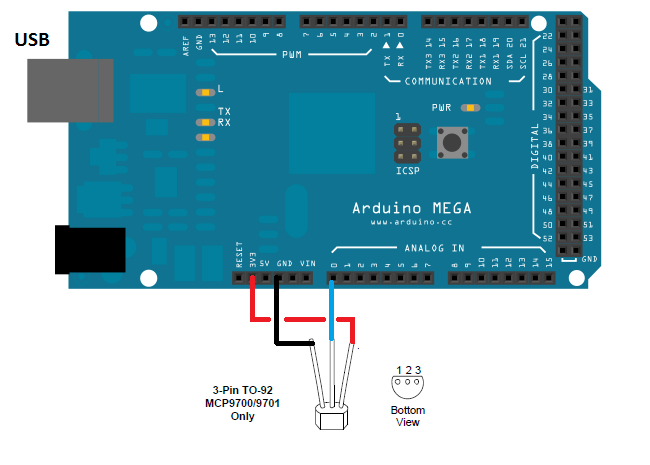# Active Sensor Thermometer © GPL3+

How to build a simple and accurate thermometer using an active temperature sensor (MCP9071/A).

• 5,371 views
• 1 comment
• 4 respects

## Components and supplies

### The Active Temperature Sensor

The project is based on the Microchip MCP9701 Active Temperature Sensor. This sensor is interfaced with the Arduino MEGA that supplies it and reads its output voltage. The MCP9701 sensor provides an output voltage that is proportional to the temperature. The sensor has a linear output characteristic defined by the formula:

`vout = tc * ta + v0 `

Where v0 is the voltage at 0°C, while tc is the temperature coefficient.

The tc for the MCP 9071 / A is 19.5 mV / °C while for the MCP9700 / A the tc is 10 mV / °C.

The output voltage vout0, for the MCP9701 / A, is 400mV. The temperature ta measured by the sensor, note the voltage vout, is:

ta = (vout-vout0) / tc

The value of Vout is obtained by averaging 1024 values reading the voltage on pin A0 that is connected to the sensor output. The value of ta will be calculated by the formula and shown on the serial monitor.

## Code

##### Arduino code for thermometer active sensor basedArduino
taking the application scheme as referencehow to connect the sensor to the Arduino board and loading this code in the arduino, it is possibile read the room temperature in the serial output interface. Every 1s the measure is done and printed
```/*

The value of Vout is obtained by averaging 1024 values reading the voltage on pin A0. whicch is connected to the sensor output. The value of temeprature ta, read by the sensor is shown on the serial monitor.

*/

// set pin numbers:

const int in = A0;            // input for reading the sensor output

// set constants:
const float vout0 = 400;   //  sensor output voltage in mV at 0°C
const float tc = 19.53;   // mV for °C temperature constant for the  MCP9701/A

// variables:

int i, f;
float vout, vout_avg, ta;

// MAIN PROGRAM

void setup() {
Serial.begin(9600);
pinMode(in, INPUT);            // set pin in as input
pinMode(13, OUTPUT);            // set pin 13 as output
digitalWrite(13, LOW);
analogReference(DEFAULT);

}

void loop() {
vout_avg = 0;
for (i = 0; i < 1024; i++) {
vout = analogRead(A0) * (4976.30 / 1023);
//Serial.println(vout);
vout_avg = vout_avg + vout;
}
vout = vout_avg / 1024;
//Serial.println(vout);
ta = (vout - vout0) / tc;
Serial.print("temperature ( celcius degree)   = " );
Serial.println(ta);
delay (1000);
}
```

## Schematics

The hardware implementation is executed by using the Arduino MEGA 2560 card: just connect the active sensor as shown in the figure. Pin A0 is set as an analog input for converting the output voltage of the sensor to the AD.April 25, 2017

#### Members who respect this project

See similar projects
you might like

by microst

• 5,975 views
• 8 respects

#### Touch Sensor and Sound Sensor Controlling AC/DC Lights

Project showcase by Anbumachi

• 3,488 views
• 7 respects

#### DIY Art Deco Analog Thermometer

Project tutorial by Nick Koumaris

• 6,452 views
• 26 respects

• 5,726 views
• 16 respects

#### Remote & Base Sensor Station - Now with IoT!

Project showcase by vapor83

• 3,663 views